# Series Rules

Series rules tell you if a series converges (settles on a certain number) or diverges (fails to settle on a number). The following table sums up the three basic rules.

 Rule # Series looks like this… Converges or diverges? To this: 1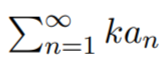Converges (if k = 0)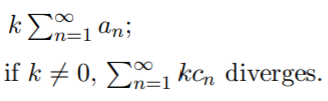2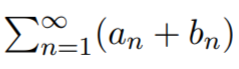Converges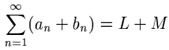3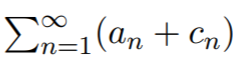Diverges (Does not converge)

## Tips on Applying Series Rules and Testing for Convergence

Half of the battle in applying series rules is figuring out which rule applies to your particular series. This graphic shows you the basic steps and tests:

Follow these steps if you aren’t sure where to start.

Step 1: Try the divergence test. The series diverges if the terms of the series don’t converge to zero.

Step 2: Look for familiar series to see if you can apply a common test.
A p-series looks like this:If you do have this type of series, it converges if p > 1, otherwise it diverges. If you aren’t sure, apply the p-series test for convergence.

If you have a geometric series, there’s also a straightforward rule: it converges if the absolute value of the common ratio, |r|, is less than 1. A geometric series is where every two successive terms have the same ratio:The geometric series converges to a/(1 – r) if it starts with n = 0.

If a series is similar to either the p-series or geometric series, you can use the limit comparison test or comparison test to determine convergence.

Other common forms to look for include the telescoping seriesand alternating series:Look out for series that are sums or multiples of familiar series before applying series rules. For example, this series converges:And this one diverges (Andrew, 2017):Step 3: If the series contains sin(x) or cos(x), try the comparison test or limit comparison test.

Step 4: Does the series contain factorials, powers, or products? Try the Ratio Test.

For a full rundown on all of the available tests, see: Series Convergence Tests

## References

Andrews, M. (2017). Math 31B: Sequences and Series. UCLA Mathematics Department. Retrieved August 7, 2020 from: https://www.math.ucla.edu/~mjandr/Math31B/series.pdf
Thomas Nelson Math Center. Rules for Testing Series Convergence. Retrieved August 7, 2020 from: https://libguides.tncc.edu/ld.php?content_id=8143534

CITE THIS AS:
Stephanie Glen. "Series Rules" From StatisticsHowTo.com: Elementary Statistics for the rest of us! https://www.statisticshowto.com/series-rules/